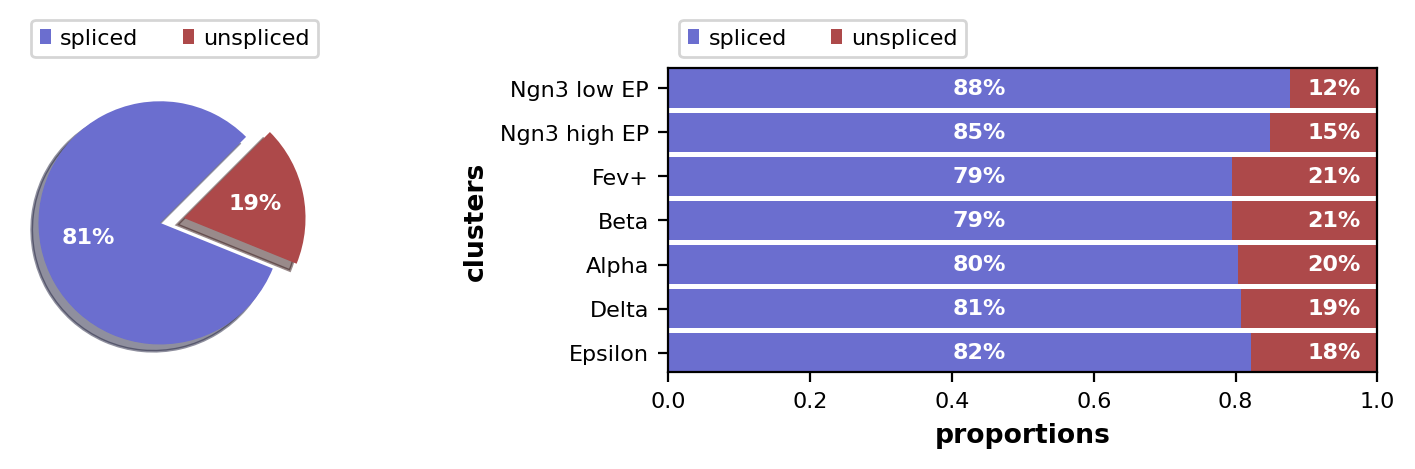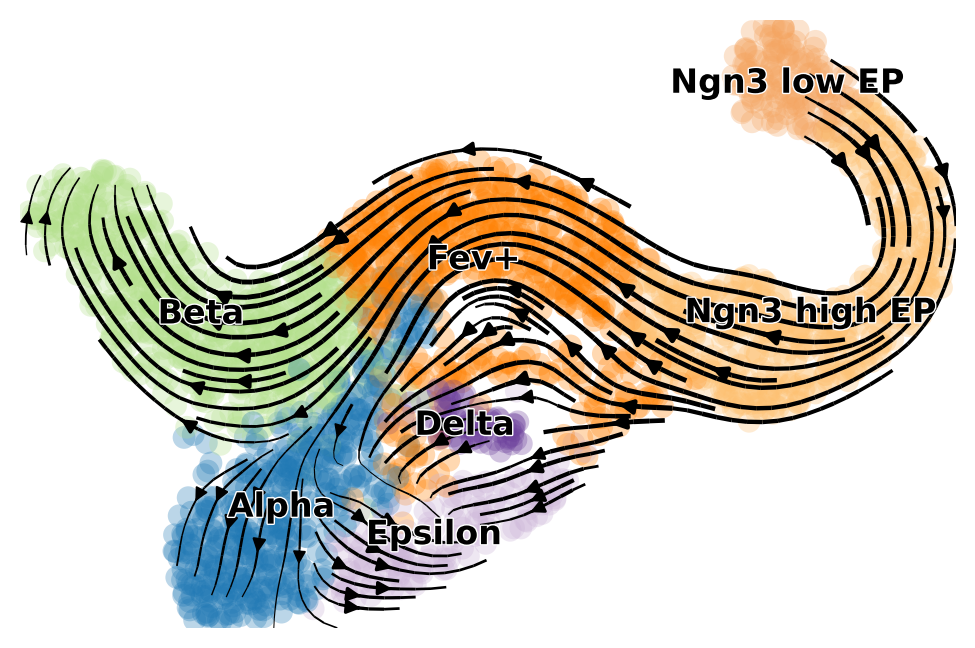# CellRank basics¶

This tutorial introduces you to CellRank's high level API for computing initial & terminal states and fate probabilities. Once we have the fate probabilities, this tutorial shows you how to use them to plot a directed PAGA graph, to compute putative lineage drivers and to visualize smooth gene expression trends. If you want a bit more control over how initial & terminal states and fate probabilities are computed, then you should check out CellRank's low level API, composed of kernels and estimators. This really isn't any more complicated than using scikit-learn, so please do check out the Kernels and estimators tutorial.

In this tutorial, we will use RNA velocity and transcriptomic similarity to estimate cell-cell transition probabilities. Using kernels and estimators, you can apply CellRank even without RNA velocity information, check out our CellRank beyond RNA velocity tutorial. CellRank generalizes beyond RNA velocity and is a widely applicable framework to model single-cell data based on the powerful concept of Markov chains.

The first part of this tutorial is very similar to scVelo's tutorial on pancreatic endocrinogenesis. The data we use here comes from Bastidas-Ponce et al. (2018). For more info on scVelo, see the documentation or read the article.

## Import packages & data¶

Easiest way to start is to download Miniconda3 along with the environment file found here. To create the environment, run conda create -f environment.yml.

In :
import scvelo as scv
import scanpy as sc
import cellrank as cr
import numpy as np

scv.settings.verbosity = 3
scv.settings.set_figure_params('scvelo')
cr.settings.verbosity = 2

In :
import warnings
warnings.simplefilter("ignore", category=UserWarning)
warnings.simplefilter("ignore", category=FutureWarning)
warnings.simplefilter("ignore", category=DeprecationWarning)


First, we need to get the data. The following commands will download the adata object and save it under datasets/endocrinogenesis_day15.5.h5ad. We'll also show the fraction of spliced/unspliced reads, which we need to estimate RNA velocity.

In :
adata = cr.datasets.pancreas()Out:
AnnData object with n_obs × n_vars = 2531 × 27998
obs: 'day', 'proliferation', 'G2M_score', 'S_score', 'phase', 'clusters_coarse', 'clusters', 'clusters_fine', 'louvain_Alpha', 'louvain_Beta', 'palantir_pseudotime'
var: 'highly_variable_genes'
uns: 'clusters_colors', 'clusters_fine_colors', 'day_colors', 'louvain_Alpha_colors', 'louvain_Beta_colors', 'neighbors', 'pca'
obsm: 'X_pca', 'X_umap'
layers: 'spliced', 'unspliced'
obsp: 'connectivities', 'distances'

## Pre-process the data¶

Filter out genes which don't have enough spliced/unspliced counts, normalize and log transform the data and restrict to the top highly variable genes. Further, compute principal components and moments for velocity estimation. These are standard scanpy/scvelo functions, for more information about them, see the scVelo API.

In :
scv.pp.filter_and_normalize(adata, min_shared_counts=20, n_top_genes=2000)

Filtered out 22024 genes that are detected 20 counts (shared).
Normalized count data: X, spliced, unspliced.
Extracted 2000 highly variable genes.
Logarithmized X.
computing moments based on connectivities
'Ms' and 'Mu', moments of un/spliced abundances (adata.layers)


## Run scVelo¶

We will use the dynamical model from scVelo to estimate the velocities. Please make sure to have at least version 0.2.3 of scVelo installed to make use parallelisation in scv.tl.recover_dynamics. On my laptop, using 8 cores, the below cell takes about 1:30 min to execute.

In :
scv.tl.recover_dynamics(adata, n_jobs=8)

recovering dynamics (using 2/2 cores)
'fit_pars', fitted parameters for splicing dynamics (adata.var)


Once we have the parameters, we can use these to compute the velocities and the velocity graph. The velocity graph is a weighted graph that specifies how likely two cells are to transition into another, given their velocity vectors and relative positions.

In :
scv.tl.velocity(adata, mode='dynamical')

computing velocities
'velocity', velocity vectors for each individual cell (adata.layers)
computing velocity graph
'velocity_graph', sparse matrix with cosine correlations (adata.uns)

In :
scv.pl.velocity_embedding_stream(adata, basis='umap', legend_fontsize=12, title='', smooth=.8, min_mass=4)

computing velocity embedding## Run CellRank¶

CellRank offers various ways to infuse directionality into single-cell data. Here, the directional information comes from RNA velocity, and we use this information to compute initial & terminal states as well as fate probabilities for the dynamical process of pancreatic development.

## Identify terminal states¶

Terminal states can be computed by running the following command:

In :
cr.tl.terminal_states(adata, cluster_key='clusters', weight_connectivities=0.2)

Computing transition matrix based on logits using 'deterministic' mode
Estimating softmax_scale using 'deterministic' mode
Setting softmax_scale=3.7951
Finish (0:00:11)
Using a connectivity kernel with weight 0.2
Computing transition matrix based on adata.obsp['connectivities']
Finish (0:00:00)
Computing eigendecomposition of the transition matrix
Adding adata.uns['eig_fwd']
.eigendecomposition
Finish (0:00:00)
WARNING: Unable to import petsc4py or slepc4py. Using method='brandts'
WARNING: For method='brandts', dense matrix is required. Densifying
Computing Schur decomposition
Adding adata.uns['eig_fwd']
.eigendecomposition
.schur
.schur_matrix
Finish (0:00:10)
Computing 3 macrostates
Adding .macrostates_memberships
.macrostates
.schur
.coarse_T
.coarse_stationary_distribution
Finish (0:00:00)
Adding adata.obs['terminal_states_probs']
adata.obs['terminal_states']
adata.obsm['macrostates_fwd']
.terminal_states_probabilities
.terminal_states
Finish


The most important parameters in the above function are:

• estimator: this determines what's going to behind the scenes to compute the terminal states. Options are cr.tl.estimators.CFLARE ("Clustering and Filtering of Left and Right Eigenvectors") or cr.tl.estimators.GPCCA (Generalized Perron Cluster Cluster Analysis). The latter is the default, it computes terminal states by coarse graining the velocity-derived Markov chain into a set of macrostates that represent the slow-time scale dynamics of the process, i.e. it finds the states that you are unlikely to leave again, once you have entered them.
• cluster_key: takes a key from adata.obs to retrieve pre-computed cluster labels, i.e. 'clusters' or 'louvain'. These labels are then mapped onto the set of terminal states, to associate a name and a color with each state.
• n_states: number of expected terminal states. This parameter is optional - if it's not provided, this number is estimated from the so-called 'eigengap heuristic' of the spectrum of the transition matrix.
• method: This is only relevant for the estimator GPCCA. It determines the way in which we compute and sort the real Schur decomposition. The default, krylov, is an iterative procedure that works with sparse matrices which allows the method to scale to very large cell numbers. It relies on the libraries SLEPc and PETSc, which you will have to install separately, see our installation instructions. If your dataset is small (<5k cells), and you don't want to install these at the moment, use method='brandts'. The results will be the same, the difference is that brandts works with dense matrices and won't scale to very large cells numbers.
• weight_connectivities: weight given to cell-cell similarities to account for noise in velocity vectors.

When running the above command, CellRank adds a key terminal_states to adata.obs and the result can be plotted as:

In :
cr.pl.terminal_states(adata)